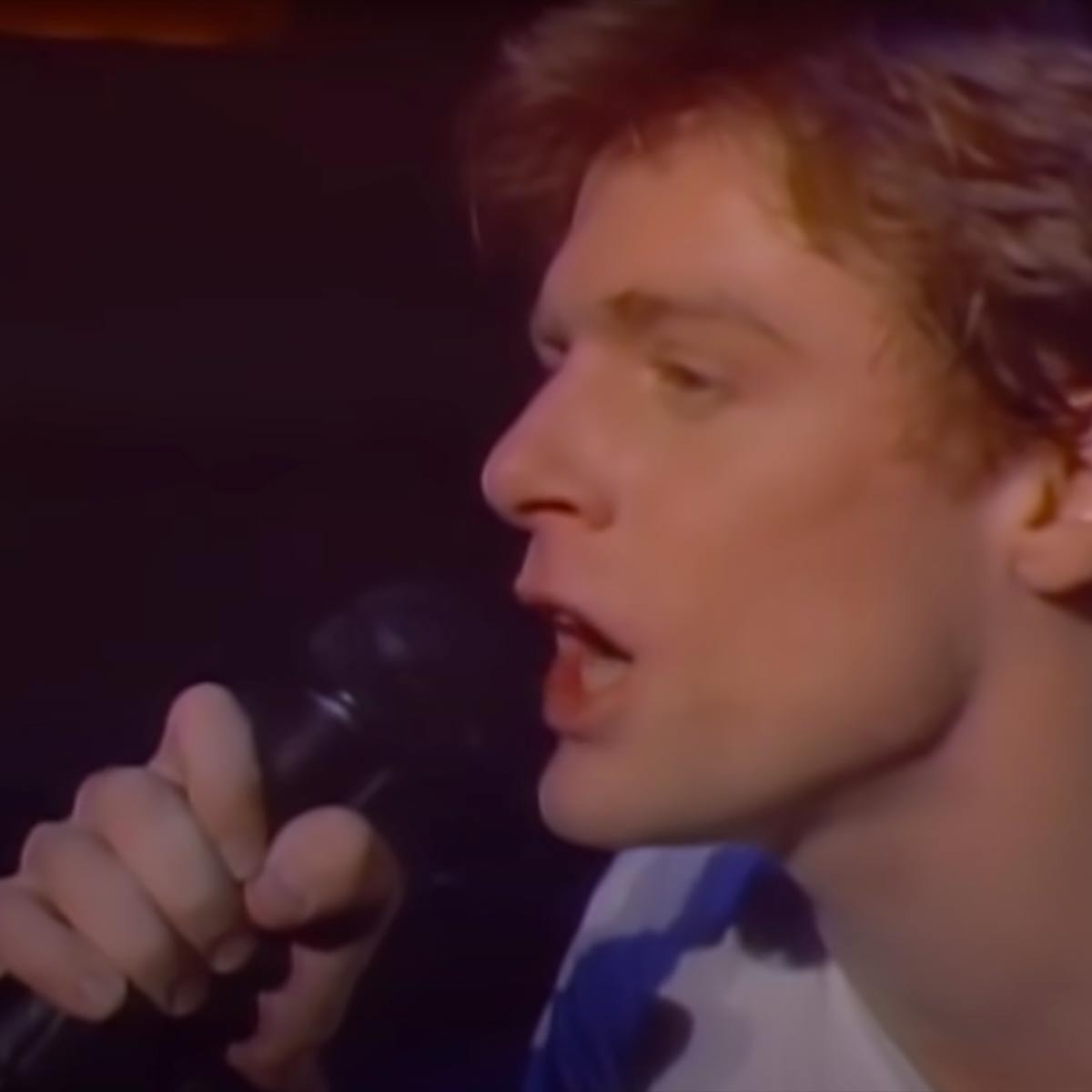# Listen To Your Heart

10 September 2020
3
• ##### TYPE
0
(0)

[e6p] s f [qh] g f dd s [ef] ashf[qs] opad[es] ap ff[qh] |
gg f dd s f |
ff[qh]gfdshhjf ds ppasp psddsf ffdsds ds j |
ffdsds psdfgf d dfdsds psdfgf d sdhhjhd dssgf |
ffdsds |
popasdsap

Rate This Music Sheet:

Average rating 0 / 5. Vote count: 0

No votes so far! Be the first to rate this music sheet.

Thank you for rating this song!

If you have any specific feedback about how to improve this music sheet, please submit this in the box below.

• A song by

## About This Music Sheet

Listen To Your Heart is a song by Roxette. Use your computer keyboard to play Listen To Your Heart on Virtual Piano. This is an Easy song and requires practice. The song Listen To Your Heart is classified in the genre of Pop Rock on Virtual Piano. You can also find other similar songs using Sweden, Love Songs.

## Related

•3 4 \$ 5 8 [f4] [p4] [f4]
[f4] j [f4] 4 s [d9] f g
[f5] d s [a0] s d [s6] u
[i9] [s9] [s9] 5 s [a5] [s5] d
p [tp] [o8] o 8 t [f0] [O0]
[f0] [f6] j [f6] 6 s [s9] d
f [d5] s [a0] s d [s6] p
[o0] [o0] [o0] [o(] [d(] [d(] [p9] [f9]
[f9] [wf] [wd] [wf] [wg] [wh] 9 q
[wh] 9 q w [h6] [j0] [wJ] [j6]
[h0] w e [g9] 6 8 [g9] 6
8 9 [qg] [h8] [H(] [h^] [qg] W
[hE] [f6] [p6] [f6] 5 [f\$] j [f\$]
\$ s [d5] 5 5 [d0] f g
[f0] 0 d f d [s4]

Level: 4
Length: 00:47
Easy

#### Shogo Sakai

•J j h g d g d g d g J j h g d g d g d g J j h g d g d g d g J j h g d g d g d g J j h g h g g d g s P o d g s P o d ss ss ss P d s P h g g d g s P J j h g d g d g d PP sds PPP sdgs P P sds P sds P sdgs PP sds P sds P sdgs PP sds P sds P sdgs P h ggg d g s P o d g s P o d ss ss ss P d s P h ggg d g s PP sds PPP sdgs P P sds P sds P sdgs PP sds P sds P sdgs PP sds P sds P sdgs P h g g d g s P o d g s P o d ss ss ss P d s P h g g d g s P J j h g d g d g d h gg d g s P o d g s P o d ss ss ss P d s P hh j J j h g J j h g d g d g d h g g d g s P o d g s P o d ss ss ss P d s P h g g d g s P J j h g d g d g d PP sds PPP sdgs P P sds P sds P sdgs PP sds P sds P sdgs PP sds P sds P sdgs P h gg d g s P o d g s sss P sss d sss P d s P PP s d s P s PPP s d s P gggg ddddddd s P P h gg d g s P oo d g s P oo d ss ss ss P d s P hh j J j h g J j h g d g d g d hh j J J J J J h h J J J J J h h h J J J J j j j h j J h h j J j h g d g d g d h g g d g s P o d g s P o d ss ss ss P d s P h g g d g s P J j h g d g d g d h g g d g s P
Level: 3
Easy

#### Lukas Graham

•h|hgff ff fdd| |psd dd fp||psd dd fd| | h|hgff ff fdd| |psd d d ss||sf f dd| | pssdd d sd| |sssd df f|| fgh hh gf s||psssd df f|| dsd a| |oss d df f|| fgh hh gf s||psssd df f|| dsd ah|hgff ff fdd| |psd dd fp||psd dd fd| | h|hgff ff fdd| |psd d d ss||sf f dd| | pssdd d sd| |sssd df f||fgh hh gf s|| psssd df f||dsd a| | oss d df f||fgh hh gf s|| psssd df f||dsd a||| dfg fd s s||sj h hghf|| fgf ddsd|||dfg f dss| | ssjh|hhfs| |h|fhj hh jfh|jsssd df f||fgh hh gf s|| psssd df f||dsd a| | oss d df f||fgh hh gf s|| psssd df f||fgh h|| lj h j j|h|fhjhhffs hjhhf d
Level: 2
Length: 02:34
Easy

#### Bryan Adams